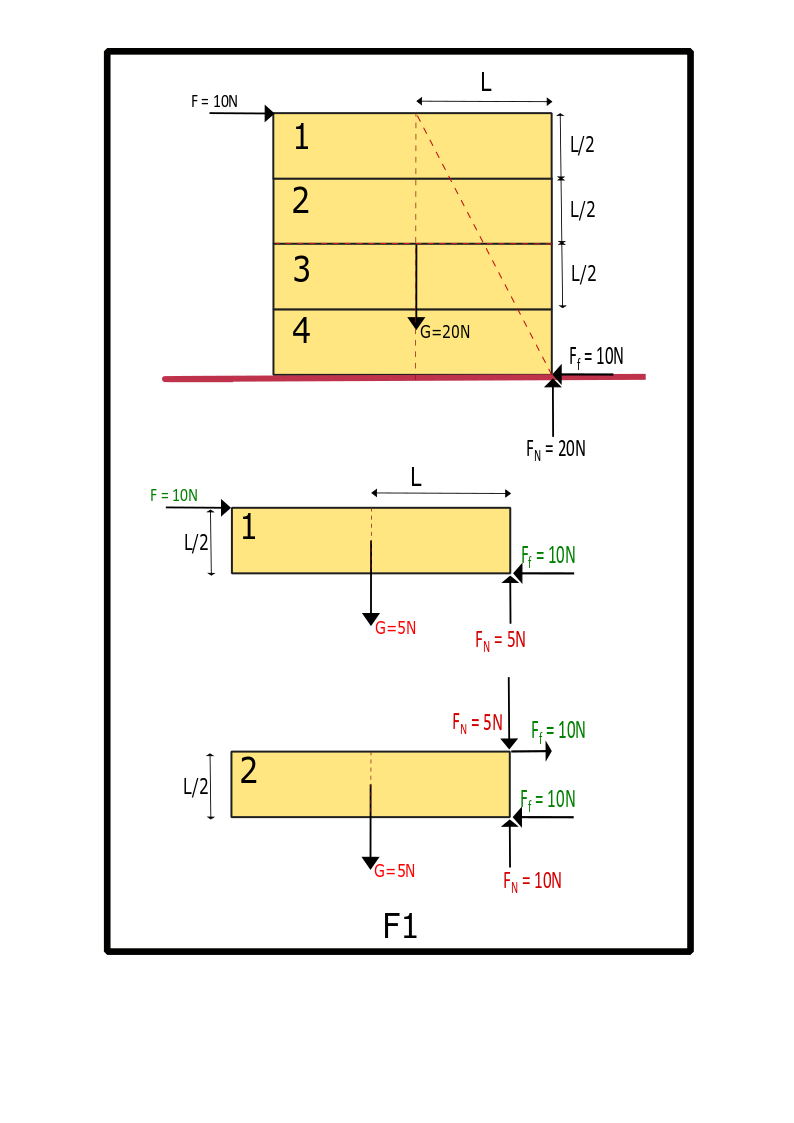# How Can We Draw The Moment Diagram Of Object 1 And 2 ? (For Example, On A Surface)

No replies to this topic

### #1civilengineer8

civilengineer8

Curious

•• Members
•• 1 posts

Posted 04 June 2020 - 06:45 PM

An identical square body (consisting of 4 boxes), all surfaces are identical and have equal friction. I calculated the loads in box 1 and 2. But how can we calculate the moment and shear force diagrams of these boxes?